International Journal of Astrophysics and Space Science
Volume 4, Issue 3, June 2016, Pages: 26-31

Formation of Planetesimals by Burgers Vortex

Martin G. Abrahamyan

Department of Physics, Yerevan State University, Yerevan, Armenia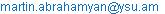Martin G. Abrahamyan. Formation of Planetesimals by Burgers Vortex. International Journal of Astrophysics and Space Science. Vol. 4, No. 3, 2016, pp. 26-31. doi: 10.11648/j.ijass.20160403.11

Received: August 23, 2016; Accepted: September 1, 2016; Published: September 21, 2016

Abstract: The effect of a Burgers vortex on formation of planetesimals in a protoplanetary disc in local approach is considered. It is shown that there is not any circular orbit for rigid particles in centrifugal balance; only stable position in Burgers vortex under the influence of centrifugal, Coriolis, pressure gradient and Stokes drag forces is the center of vortex. The two-dimensional anticyclonic Burgers vortex with homogeneously rotating kernel and a converging radial stream of substance can effectively accumulate in its nuclear area the meter-sized rigid particles of total mass ~1028g for characteristic time ~ 106yr.

Keywords: Protoplanetary Disc, Burgers Vortex, Planetesimals

Contents

1. Introduction

The radial dependence of infra-red, sub mm and cm radiation of protoplanetary disks analyses show that vortices serve as incubators for growth dust particles and formation of planetesimals . Formation of planetesimals from micron size dust grains possibly, involves many physical processes . It is accepted that growth of particles in dusty circumstellar discs is hierarchical [3, 4]. The initial stage of growth probably proceeds through the nucleation of sub-micron-sized dust grains from the primordial nebula, which then forms the monomers of fractal dust aggregates up to ~ 1mm to ~ 10cm for characteristic time of an order of 103 years. The further growth of particles by this mechanism is stopped by collisional destruction process [5, 6]. In this stage the dynamics of particles and coagulation are regulated by van der Waals forces and the Brownian motion. The best astrophysical evidence for grain growth to specified sizes is the detection of 3.5 cm dust emission from the face-on disk of radius 225 AU round classical T Tauri star TW Hya (age ~ 5-10 Myr), located 56 pc away . When the planetesimals reached a size of about 1 km, they began to attract other smaller bodies due to their gravity.

In standard models of protoplanetary disks, gas pressure decreases along radius. Gas in a disk practically moves on sub-Keplerian speeds. Rigid particles, under the action of a head wind drags, lose the angular momentum and energy. As a result, the ~10 cm to meter-sized particles drift to the central star for hundreds of years, that is, much less than the lifetime of a disk which makes several millions of years [8, 9].

Long-lived vortical structures in gas disk are a possible way to concentrate the ~10 cm to meter sized particles and to grow up them in planetesimal. Similar effect of vortices on the Earth observed in special laboratories, and also in the ocean. For example, the oceanic vortices trap larval fish off the coast of Western Australia .

In some areas of the stratified protoplanetary disks the current has 2D-turbulent character. An attractive feature of such hydrodynamic current consists in the fact that in it, through a background of small whirlpools, long-living vortices will spon­taneously be formed without requirement of special initial conditions [11-13]. In laboratory experiments , formation of Burgers vortex which will be considered in the present work is often observed in 2D-turbulent flows. If protoplanetary disks are capable of producing 2D-turbulent flow, they can form long-living large-scale vortices which in due course lives up to an order of hundred orbital periods. Anticyclonic vortices in a protoplanetary disk merge with each other and amplify, while cyclonic ones are destroyed by a shear flow.

In an anticyclone, rigid particles are grasped by force of Coriolis directed to the center of a vortex. If the vortex survives ~ 100 rotations in a nebulae with solar mass, the quantity of the grasped particles can reach masses of planets (several masses of the Earth). Existence of long-living vortices in the protoplanetary disk, drifting of external regions of a disk, allows accumulation of the mass necessary for formation of a kernel of a giant planet [15, 16].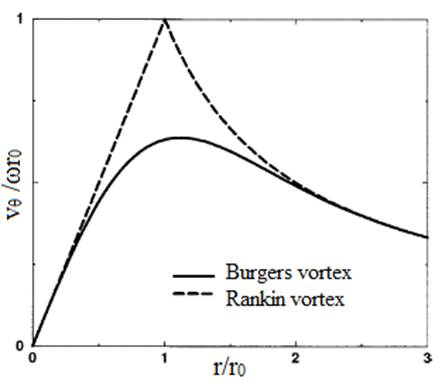Fig. 1. Rotational velocity profiles of Burgers and Rankin vortices.

In the present work the Burgers vortex in a protoplanetary disk and its role in a problem of formation of planetesimals will be considered.

The vortex of Burgers in cylindrical system of co-ordinates (r, θ, z) is defined as

vr = - Αr, vθ = ωr02 [1- exp (-r2/r02)]/r, vz = 2Αz.    (1)

This represents a vortex with a converging stream of substance to its center where Α characterizes a converging stream, and ω and r0 - circulation and the size of a trunk of a vortex. Rotation in the field of a vortex trunk is almost rigid-state and on the big distances a profile of rotational speed falls down under the hyperbolic law (fig. 1). The asymptotic behavior of Burgers vortex in small and big distances from the vortex center represents the Rankin vortex [17,18]. The maximum rotational speed in Burgers vortex is equal 0.638ωr0 which is reached at r/r0 = 1.121. At distance reff = 4.5 r0, rotational speed makes one-third of the maximum value. Conditionally we will name this distance "effective radius" of Burgers vortex.

2. The Magnitude of Some Parameters of a Disk

We consider the Burgers vortex in viscous axially-symmetrical accretion disk with effective temperature T, gas volume density ρ, of almost Keplerian rotation. The sound speed in gas is estimated by the formula

cs = √γkT/mH ≈ (γT/100K)1/2km/s,       (2)

where γ =1.4 – the gas adiabatic index, k - Boltzmann constant, mH - hydrogen atom mass.

In a vertical direction, the gas is in hydrostatic balance with a characteristic scale height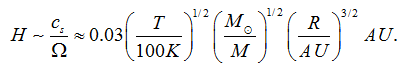(3)

The superficial density of the gas in a disk can be estimated as Σ 2H ρ.

In α - model of accretion disk , the expense of gas occurs with a speed dm/dt = 3πνΣ, where ν - kinematic viscosity of gas - ν = α cs H.

The characteristic dynamic time scale of a disk is: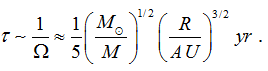(4)

For Keplerian disk, radial momentum equation solution yields to a difference between the speeds of rigid particles and surrounding gas . In a thin gas disk (cs ΩR), rigid particles drift relative to gas with a speed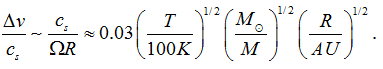(5)

At cs ~ 1km/s, typical drift speed is of order 30 m/s. The characteristic scale of drift time [8, 9] almost by two orders surpasses dynamic time τ

τd ~ r / ∆ v ~ (R/A.E.) 102 yr.         (6)

Dust settled on a midplane of a disk for a characteristic time 

τs ~ Σ / αΩρ *,                (7a)

where ρ* is the mass density of a particle, and characteristic time between collisions of rigid particles among themselves is estimated as

τcol ~ Dρ* / Σ *Ω,              (7b)

where D is the diameter of a particle, Σ* is the superficial density of rigid particles in a disk which is more than by two orders less than a disk Σ. For metre-size rigid particles, this time is an order ~ 5yr. There­fore for creation of rigid particles with the sizes significantly surpassing the meter size, it is necessary to provide an environment with high concentration of particles in rather small regions. Such environment is provided by vortices.

Particles in a vortex are exposed to actions of centrifugal, Coriolis, drag forces, and in a smaller measure, to gradient forces of pressure. If centrifugal force deletes a particle from the vortex centre, drag and Coriolis forces in an anticyclonic Burgers vortex are directed to its centre. Acceleration of a rigid particle is directed to the vortex centre if the vortex angular speed ω = |d θ/dt | at a small friction is less than 2Ω,

ω < 2Ω                    (8)

- a condition which is always carried out in practice.

Viscous dissipation and orbital shear limit the sizes of a vortex. Viscous dissipation destroys vortices of sizes less than viscous scale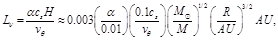(9)

where vθ - rotational speed of a vortex.

The Keplerian shear flow forbids formation of circular structures with the sizes larger than shear length scale ,

Lshear =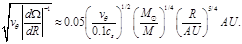(10)

The vortices, whose sizes surpass Lshear, are extended in an azimuthal direction that allows them to survive longer. In  we have shown the possibility of formation in a disk extended in an azimuthal direction three-axis ellipsoidal vortex, with a linear field of circulation, similar to Riemann S ellipsoids . However, note that in a disk round the central star of solar mass, at distance 30AU, the vortex of characteristic speed of rotation 0.01cs, can be circular and have the size of an order ~ 1AU.

In a gas disk, drag force on rigid particles from gas is exposed, which, depending on size of a particle, is expressed either by Stokes, or Epstein's formula (see, for example, ). If the sizes D particles are small in comparison with gas mean free path, such particles are exposed to the Epstein drag force: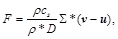(11)

where v - velocity of gas, u - velocity of rigid particle. Particles of the big sizes are exposed to the Stokes drag force (see (15)).

3. The Burgers Vortex in Local Frame of Reference

Let's use local approach, choosing frame of reference, rotating with a disk with angular speed Ω0 at distance R0 round the central star of mass M. In this approach, assuming the sizes of a vortex are much smaller than the distance R0, we will choose Cartesian system of co-ordinates with center O (Fig. 2), directing the Y axis to a star, and the X axis in direction of Keplerian flow of gas. We will present the disk rotation as Ω(R) R-q. In case when only the gravitation of the central star operates, rotation will be Keplerian with q = 3/2, and for homogeneously rotating disk q = 2, i.e. 2 ≥ q ≥ 3/2.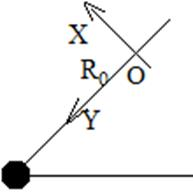Fig. 2. Local frame of reference.

In the chosen system of reference, the substance stream has X component of speed -iqΩ0y, centrifugal force is compensated by radial component of gravitation of the central star at distance R0, in other points their sum gives the tidal force j3Ω02y. The vertical component of gravitation -Ω02z is returning force along axis Z.

At first we will consider a gas disk round the central body. In local approach, the equation stationary isentropic shear flow of gas taking into account viscosity will be described by Navier-Stokes and continuity equa­tions

(v)v = j3Ω02y - kΩ02z - 2Ω0×v - h + ν∆v     (12)

(ρv) = 0,                  (13)

where h - specific enthalpy (h = ∫ρ-1dp), i, j and k - Cartesian orts. The first term in the right side of equation (12) is, as noted above, tidal acceleration in a plane of the disk, the second term is vertical component of gravitation, the third is acceleration of Coriolis, the last is a viscous stress.

In the chosen Cartesian co-ordinate system the Burgers vortex will be presented in the form

vx = - Αx - ωr02y [1- exp (-r2/r02)]/r2,

vy = - Αy + ωr02x [1- exp (-r2/r02)]/r2,      (14)

vz = 2Αz,

where r2 ≡ x2 + y2.

It is easy to check that solutions (14) satisfy to the continuity equation at dρ/dt = 0 - that is comprehensible in local approach.

Substituting (14) in the equation (12), we will receive expression for specific enthalpy h(x, y, z). However we will not present the bulky expression for h(x, y, z). We will only describe spatial profiles of gradient forces ∂h/∂x and ∂h/∂y in X, Y plane in anticyclonic Burgers vortex (fig. 3).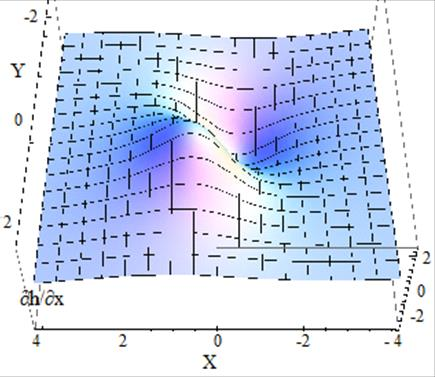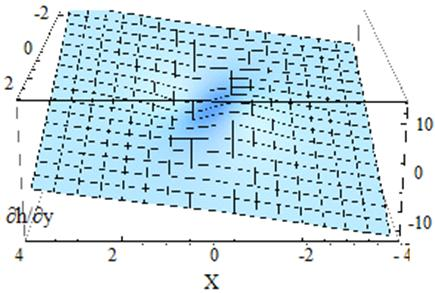Fig. 3. Profile of pressure gradient force h which in an anticyclonic Burgers vortex forces to rotate disc matter clockwise.

4. The Dynamics of Rigid Particles in Burgers Vortex

In the present work, we will be limited to studying two-dimensional dynamics of dust rigid particles in a Burgers vortex taking into account action pressure gradient force h, tidal force, forces of Coriolis and a friction. Thus we will neglect the influence of rigid particles on dynamics of gas, and also the interaction of rigid particles among themselves.

Let's consider that the sizes of particles D considerably surpass the mean free path of molecules of gas, therefore we will describe the friction of rigid particles with gas by Stokes drag force

f = β(v - u),                (15)

where

β18 ρν/ρ*D2,               (16)

u - velocity of a particle:

u = (dX/dt, dY/dt)              (17)

X, Y - particle co-ordinates.

The equation of motion of dust particles in the accepted approach looks like:

dux/dt = 2Ω0uy - β(vx|r = (X, Y) - ux) - ∂h/∂ x|r = (X, Y),(18)

duy/dt = 3Ω02Y - 2Ω0ux - β (vy|r = (X, Y) - uy) - ∂h/∂y|r = (X, Y). (19)

It is convenient to present these equations in a dimensionless form. As characteristic length of a problem we will accept the size of a trunk of a vortex r0, for characteristic time and speed - 1/Ω0 and Ω0r0 respectively. Then the equations (18), (19) will become

dux/dt = 2uy + γ (vx|r = (X, Y) - ux) - ∂h/∂x|r = (X, Y),    (20)

duy/dt = 3y - 2ux + γ (vy|r = (X, Y) - uy) - ∂h/∂y|r = (X, Y),  (21)

where γ - dimensionless parameter

γ = β/Ω0 = 18 ρν/ρ*D2Ω0.           (22)

Let's consider at first the dynamics of particles in the vortex trunk (r2/r02<1) where the profile of rotation of a vortex has uniform character. In a dimensionless form

vx = - Αx - ωy + O(r2/r02), vy = - Αy + ωx + O(r2/r02).  (23)

where Α and ω are measured in units Ω0. From (2) taking into account (13) it is found

∂h/∂x = - (Α2 - ω2 - 2ω) x - 2 Α (ω + 1)y,   (24)

∂h/∂y = 2Α(ω + 1) x - (3 + Α2 - ω2 - 2ω)y.   (25)

Substituting (23) - (25) in the equations (20) and (21), we will receive the equations of motion of rigid particles in the field of a vortex trunk: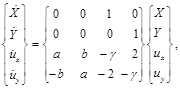(26)

where

a = A (A - γ) - (ω +1) 2 + 1; b = 2 Α (ω + 1) - γω.  (27)

From these equations it is visible that equilibrium position of rigid particles in a vortex trunk is its centre X = Y = 0, where ux = uy = 0 and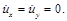Particles in considered area gradually come nearer to the centre of the vortex by helicoidal trajectories.

For establishing the stability of this position of balance, it is necessary to require real parts of eigenvalues of a matrix (26) to be zero or negative.

The matrix of factors (26) has complex eigenvalues of a kind

Λ1,2,3,4 = - γ/2 ∓ ⅈ ± √ [𝑎-1 + γ 2/4 ± (𝑏 - 𝛾)],   (28)

After allocation of real part of (28), for stability we receive a condition

(b - γ) 2 + γ2 (a - 1) ≤ 0,         (29)

at which centrifugal force is always less than the sum of drag and Coriolis forces, and the resultant force operating on a rigid particle, is directed to the vortex centre.

From (29) the necessary condition of stability follows: a ≤ 1, which taking into account (27), gives (ω +1)2> A(A - γ) that is satisfied for any positive values of A and γ. The condition (29) with the account (27) leads to stability criterion

γ > Α,                  (30)

that taking into account (16), in a dimensional form, for viscosity ν gives

ν > ρ*АD2/18ρ.              (31)

Consider now the question whether there exists an anticyclonic orbit in the volume of Burgers vortex (1) on which the sum of Coriolis and drag forces is counterbalanced by centrifugal force, i.e. in local cylindrical system of co-ordinates

γAr + 2uθ = uθ2/r, or (uθ/r) 2 - 2 (uθ/r) - γA = 0.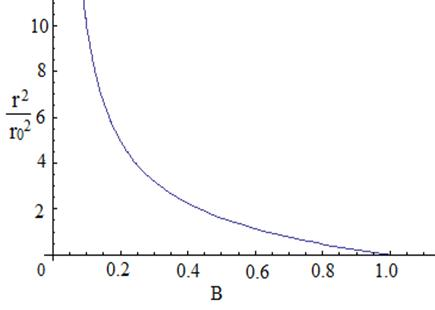Fig. 4. On the solution of transcendental equation (32).

It represents the transcendental equation which in a dimensional form looks like

[1 - exp (-r2/r02)] r02/r2 = B,          (32)

where

B [1 + √(1 + βA/Ω02)] Ω0 /ω.

The equation (32) has real solutions for orbit radius only at B≤1 (fig. 4), and r = 0 at B = 1. From expression B it is visible that B > 2Ω0/ω. This condition leads to the result B > 1. Hence, unique position of balance for rigid particles in a Burgers vortex is its centre where all particles captured by a vortex will gather during the characteristic time

τ ~ ωreff/A√βν.               (33)

The mass of the rigid particles captured by a vortex is an order

Mp ≈ π reff2Σ*,               (34)

which forms a planetesimal.

5. Discussion

Let's estimate an order of magnitudes τ and Mp for a model of a disk of radius 30AU and mass 0.5 M round a star of solar mass: M ≈ M, also we will place the local frame of reference at distance R0 = 20 AU. This gives estimations Ω0 ≈ 8·10-9s-1 and Σ ~ 1600g/cm2.

For a typical protoplanetary disk at distance R0 = 20AU. The vertical scale height is of order H ≈ 108km. For sound speed, then, we receive an estimation cs ≈ HΩ0 ≈ 0.8 km/s.

Choosing different values for vortex parametres, it is possible to receive different results. For example, accepting the maximum speed of rotation of a vortex 0.01km/s at distance r0 ≈ 107km from its center, and speed of a converging stream vr = A·r0 ≈ 0.005 km/s, we will receive

ω ≈ 10-9s-1, A ≈ 5·10-10s-1.           (35)

The condition of stability of position of rigid particles in the center of a Burgers vortex for viscosity (31) is carried out with a large supply for protoplanetary disks. The matter is that the molecular viscosity of gas, estimated by the formula ν ~ λcs, in which λ is the mean free path of molecules, сs is the speed of a sound, does not play an appreciable role in processes of a protoplanetary disk. Really, the mean free path is defined as λ ~ 1/nσ, where n is the concentration of molecules of gas, σ is the section of their interaction. Concentration of molecules is equal in the central plane of a disk to n ~ Σ/(2mH H) 1014 cm-3. Taking interaction section close to the area of a molecule of hydrogen (σ ~ 10-16 cm2), we will receive: λ ~ 20 cm, ν ~ 106cm2/s. Characteristic time of evolution of the disk is of the order of τ = R2/ν, that makes ~1013 yrs, which is 106 times more than the observable time of disk evolution.

For this reason, the ‘α – disk’  model is used to describe accretion disks, in which turbulent viscosity is represented by the expression ν ~ αcsH ≈ αH2Ω0, the dimensionless parameter α is considered as constant value of an order α ~ 10-2. The scale of viscous length thus makes L ν ≈ 106km, so Burgers vortex of big sizes cannot be destroyed by viscosity. Keplerian shear length makes Lshear ≈ 6·109km. Hence, vortices with the sizes reff < Lshear can have circular form.

Taking into account that ρ*/ρ ≈ 1010 in a midplane of a disk, using in (33) also the average value for viscosity from stability condition (31), we will receive the following estimations:

Mp ≈ 1028g; τ ~ 3·106(m/D) yrs.      (36)

So, during an order of ~106 yrs, for meter-sized rigid particles, in the vortex trunk the mass amount comparable with mass of Venus accumulates.

6. Conclusion

From stated above it follows that the formation of planetesimals by means of an anticyclonic Burgers vortex is effective enough. Operating on rigid particle in such a vortex force of Coriolis, Stokes drag force and pressure gradient force are directed to its center. There is not any circular orbit in centrifugal balance in Burgers vortex in local approach. Only stable position for rigid particle is the vortex center.

Our estimations for a typical protoplanetary disc show that the two-dimensional anticyclonic Burgers vortex with homo­geneously rotating kernel and a converging radial stream of substance can effectively accumulate in its nuclear area meter- sized rigid particles of total mass ~1028g (or more) for characteristic time ~106yr.

Acknowledgment

I am grateful to reviewer for his/her helpful suggestions

References

1. Kevin Heng, Scott J. Kenyon (arXiv:1005.1660v3 [astro-ph. EP] 17 Lul 2010).
2. Youdin, A.N., European Astronomical Society (EAS) Publication Series, 41, 187, 2010.
3. Armitage, P.J., arXiv:astro-ph/0701485v2, 2007.
4. Armitage, P.J., Astrophysics of Planet Formation, Cambridge University Press, UK, 2010.
5. Blum, J., Wurm, G., ARA&A, 46, 21, 2008.
6. Zsom, A., Ormel, C.W., Guttler, C., Blum, J., Dullemond, C.P., arXiv:1001.0488v1, 2010.
7. Wilner, D.J., D’Alessio, P., Calvet, N., Claussen, M.J., Hartmann, L., ApJ, 626, L109, 2005.
8. Adachi, I., Hayashi, C., Nakazawa, K., Prog. Theor. Phys., 56, 1756, 1976
9. Weidenschilling, S.J., MNRAS, 180, 57, 1977.
10. Paterson, H.L., Feng, M., Waite, A.M., Gomis, D., Beckley, L.E., Holliday, D., Thompson, P.A., Journal of Geophysical Research,113, C7, C07049, 2008.
11. Carnevale, G.F., McWilliams, J.C., Pomeau, Y., Weiss, J.B., Young, W.R., Phys. Rev. Lett., 66, 2735, 1991.
12. Weiss, J.B., McWilliams, J.C., Phys. Fluids A, 5, 3, 1993.
13. Tabeling, P., Physics Reports, 362, 1, 2002.
14. L&mro, B. J., Lasheras, J. C., J. Fluid Mech. 235, 179, 1992.
15. Inaba, S., Barge, P., Daniel, E., Guillard, H., A&A, 431, 365, 2005
16. Inaba, S., Barge, P., Ap. J., 649, 415, 2006
17. Abrahamyan, M.G., Astrophysics, 51, 201, 2008,
18. Abrahamyan, M.G., Matveenko, L.I., Astrophysics, 55, 443, 2012.
19. Shakura, N.I., Sunyaev, R.A., A&A, 24, 337, 1973.
20. Pringle, J. E., ARA&A, 19, 137, 1981.
21. Chiang, E.I., Goldreich, P., ApJ, 490, 368, 1997.
22. Godon, P., Livio, M., ApJ, 537, 396, 2000.
23. Abrahamyan, M.G., Astrophysics, 59, 309, 2016.
24. Chandrasekhar, S., Ellipsoidal Figures of Equilibria, Yale University Press, 1969.

 Contents 1. 2. 3. 4. 5. 6.
Article ToolsAbstractPDF(588K)